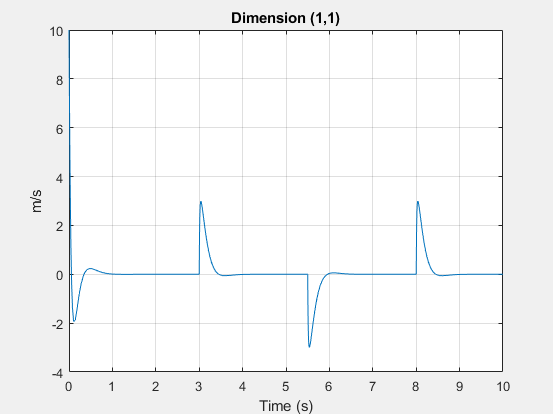# simscape.logging.Series

Time-value series for simulation data

## Description

`simscape.logging.Series` represents simulation data for a variable in a model. The series is a representation containing time-value pairs for each simulation step. The size of the series is determined by the number of simulation steps. You can also limit the size by specifying the maximum number of logged steps when you set your data logging preferences.

Final nodes in the data logging tree correspond to all the variables logged for the model. Final nodes do not have children nodes, and contain the series data logged during simulation. To specify a `Series` object, start with a full identifier path from the workspace log variable name to the name of the variable node associated with the series, and conclude with `.series`. For example, `simlog.Translational_Spring.v.series` is the series containing the simulation data for the variable `v` (velocity) of a Translational Spring block at the top level of a block diagram, given the default workspace variable name, `simlog`.

## Creation

This object is created automatically during simulation, as part of the simulation log workspace variable, if you enable data logging for the model.

## Properties

expand all

Size, or number of steps, in the simulation series.

Dimension of variable represented by the series.

The default unit associated with the values in the series.

Thermal unit conversion type (absolute or relative). For more information, see Thermal Unit Conversions.

## Object Functions

 `plot` Plot logged simulation series values against time `plotxy` Plot two series against each other `time` Extract time vector from simulation series `values` Extract values vector from simulation series

## Examples

collapse all

Plot velocity of port R of a Translational Spring block.

Open the Mass-Spring-Damper with Controller example model:

`openExample('simscape/MassSpringDamperWithControllerExample')`

This example model has data logging enabled for the whole model, with the Workspace variable name parameter set to `simlog_MassSpringDamperWithController`.

Simulate the model to log the simulation data:

`sim('MassSpringDamperWithController');`

Plot velocity of port R of the Translational Spring block `Spring`.

`plot(simlog_MassSpringDamperWithController.Spring.R.v.series);`## Version History

Introduced in R2010b